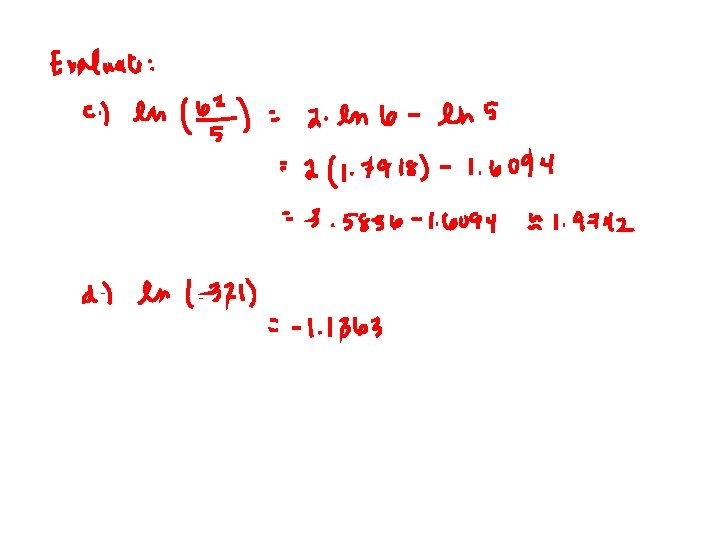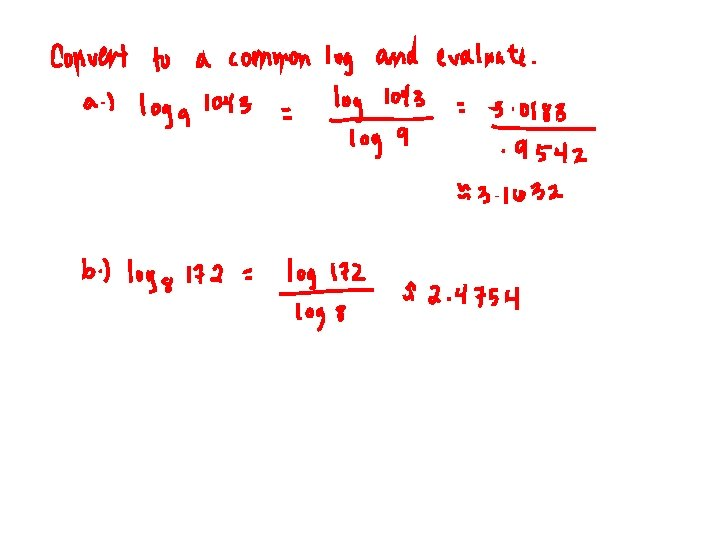# Common and Natural Logarithms Common Logarithms A common

• Slides: 22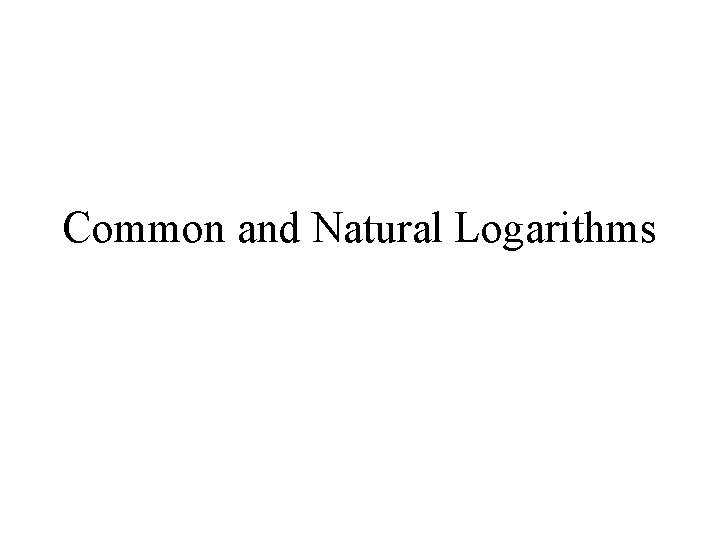Common and Natural Logarithms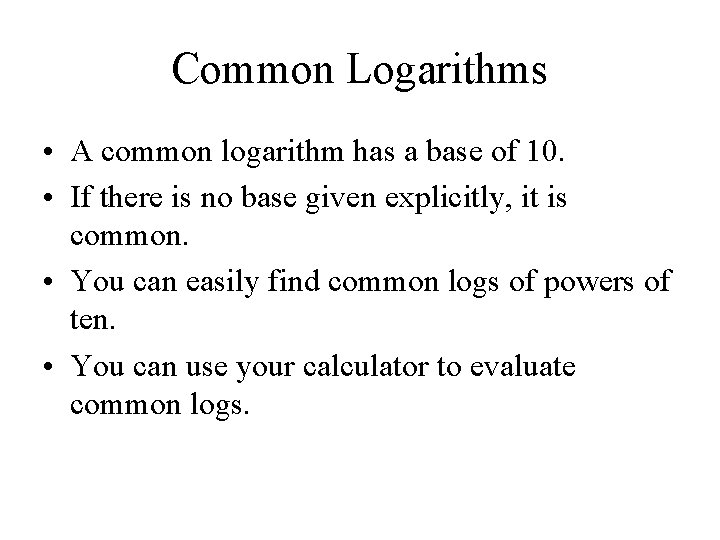Common Logarithms • A common logarithm has a base of 10. • If there is no base given explicitly, it is common. • You can easily find common logs of powers of ten. • You can use your calculator to evaluate common logs.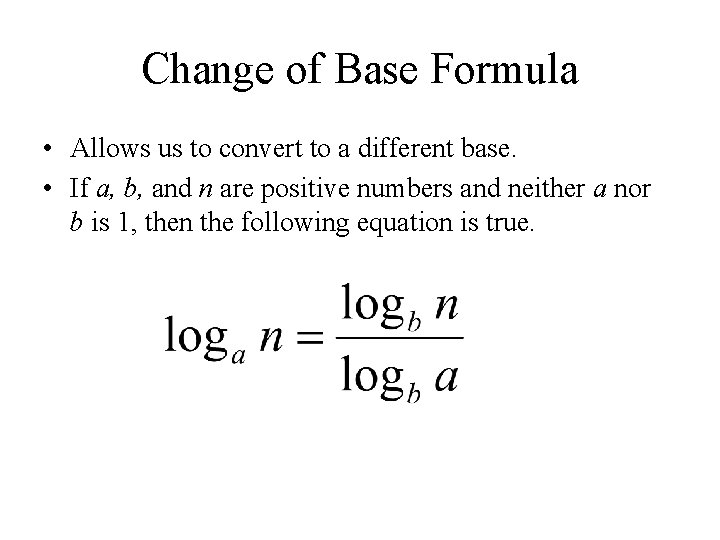Change of Base Formula • Allows us to convert to a different base. • If a, b, and n are positive numbers and neither a nor b is 1, then the following equation is true.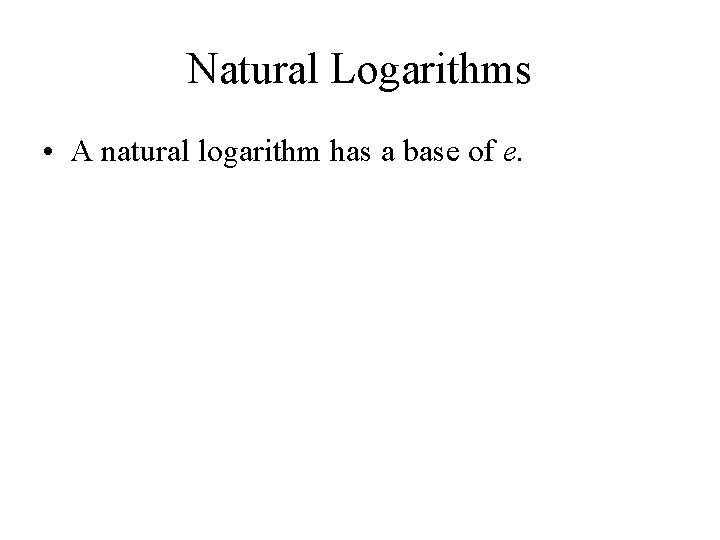Natural Logarithms • A natural logarithm has a base of e.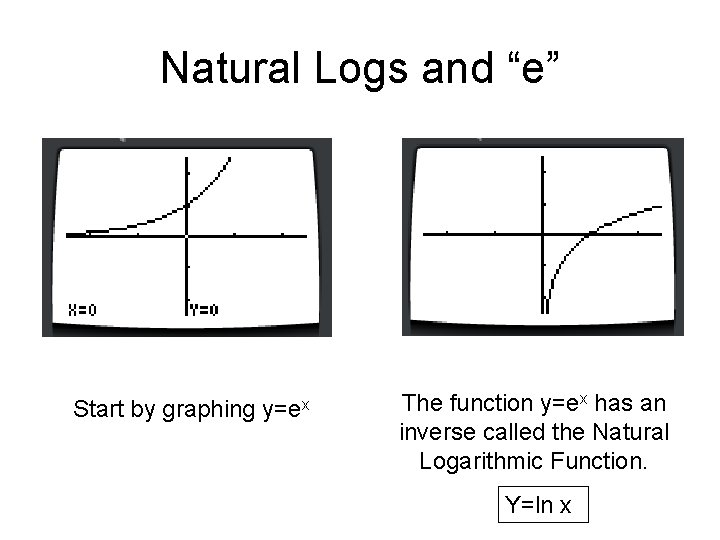Natural Logs and “e” Start by graphing y=ex The function y=ex has an inverse called the Natural Logarithmic Function. Y=ln x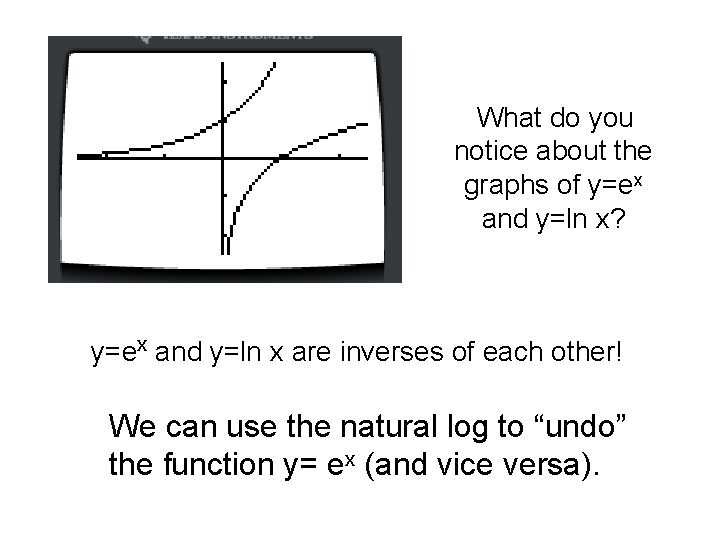What do you notice about the graphs of y=ex and y=ln x? y=ex and y=ln x are inverses of each other! We can use the natural log to “undo” the function y= ex (and vice versa).All the rules still apply • You can use your product, power and quotient rules for natural logs just like you do for regular logs Let’s try one: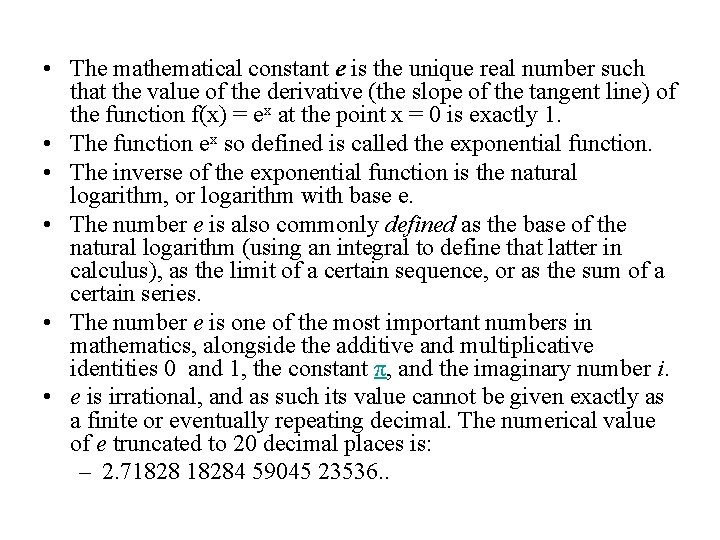• The mathematical constant e is the unique real number such that the value of the derivative (the slope of the tangent line) of the function f(x) = ex at the point x = 0 is exactly 1. • The function ex so defined is called the exponential function. • The inverse of the exponential function is the natural logarithm, or logarithm with base e. • The number e is also commonly defined as the base of the natural logarithm (using an integral to define that latter in calculus), as the limit of a certain sequence, or as the sum of a certain series. • The number e is one of the most important numbers in mathematics, alongside the additive and multiplicative identities 0 and 1, the constant π, and the imaginary number i. • e is irrational, and as such its value cannot be given exactly as a finite or eventually repeating decimal. The numerical value of e truncated to 20 decimal places is: – 2. 718284 59045 23536. .Natural Logarithms • A natural logarithm has a base of e. • We write natural logarithms as ln. – In other words, loge x = ln x. • If ln e = x…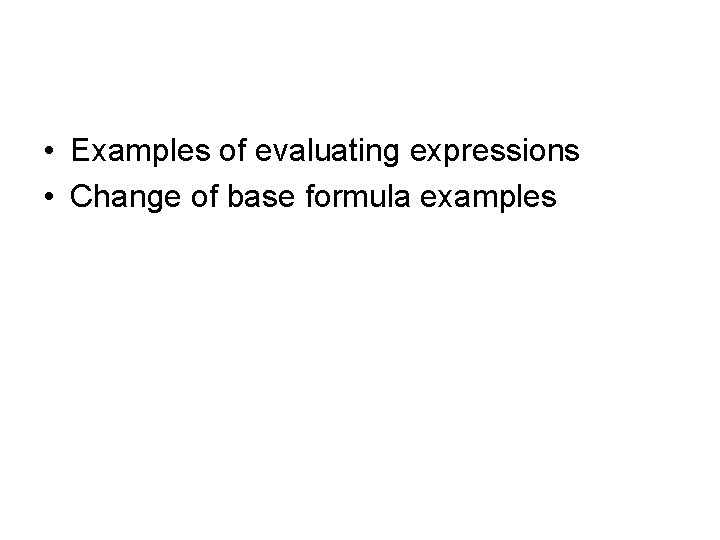• Examples of evaluating expressions • Change of base formula examples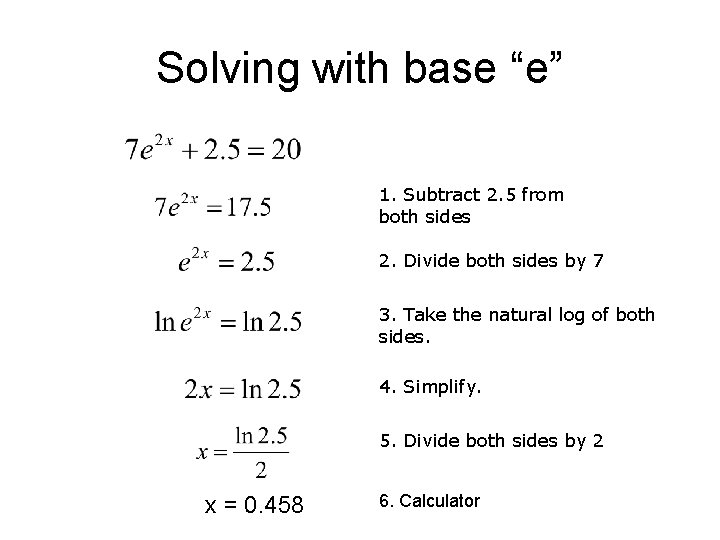Solving with base “e” 1. Subtract 2. 5 from both sides 2. Divide both sides by 7 3. Take the natural log of both sides. 4. Simplify. 5. Divide both sides by 2 x = 0. 458 6. Calculator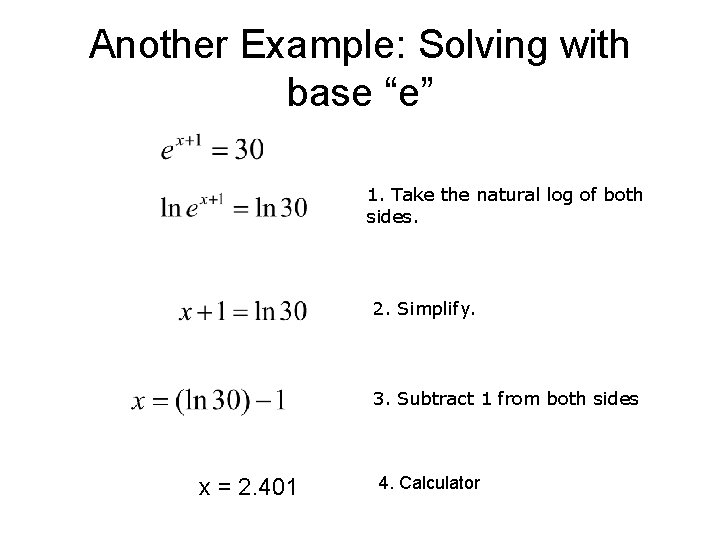Another Example: Solving with base “e” 1. Take the natural log of both sides. 2. Simplify. 3. Subtract 1 from both sides x = 2. 401 4. Calculator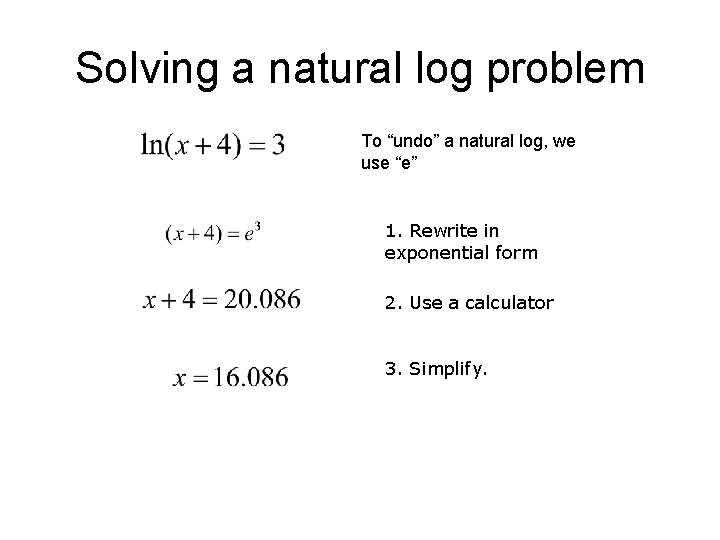Solving a natural log problem To “undo” a natural log, we use “e” 1. Rewrite in exponential form 2. Use a calculator 3. Simplify.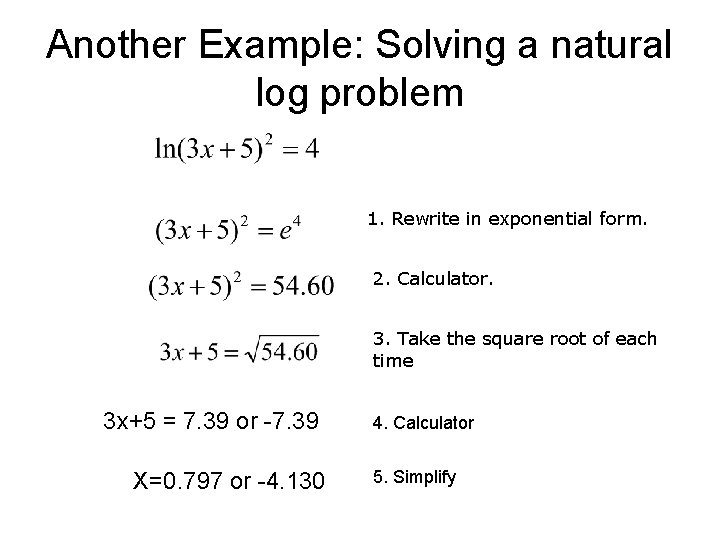Another Example: Solving a natural log problem 1. Rewrite in exponential form. 2. Calculator. 3. Take the square root of each time 3 x+5 = 7. 39 or -7. 39 X=0. 797 or -4. 130 4. Calculator 5. Simplify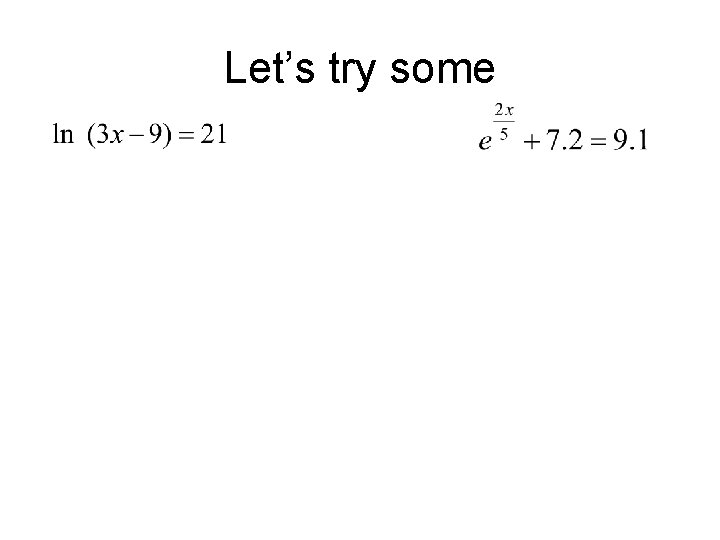Let’s try some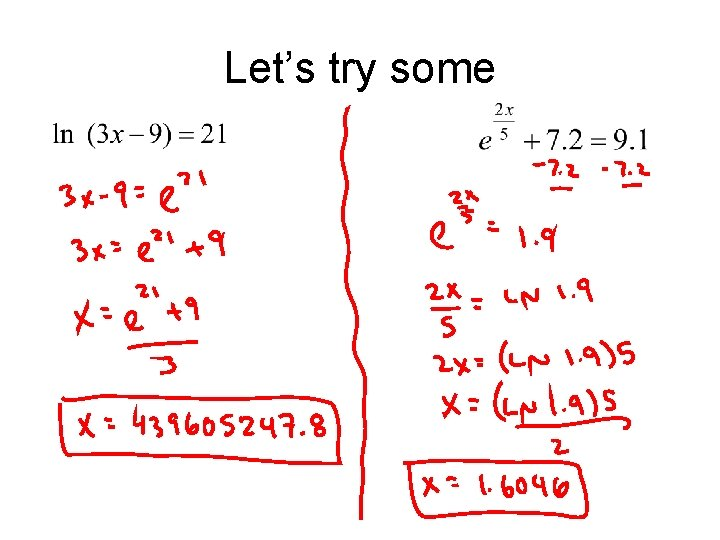Let’s try some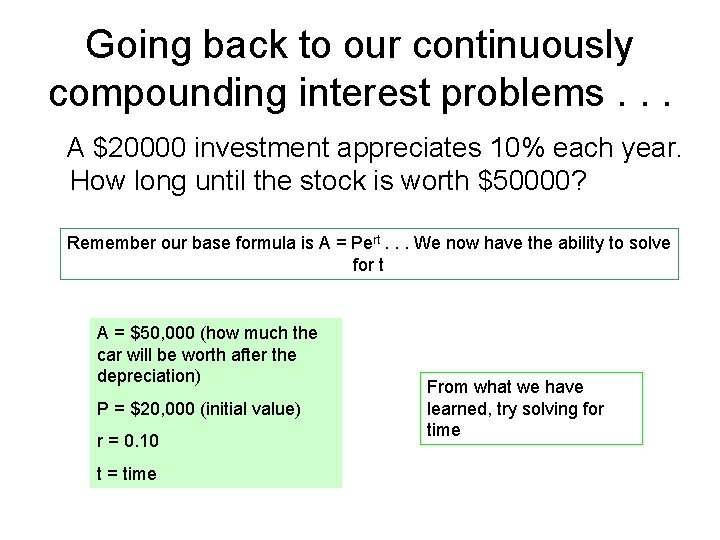Going back to our continuously compounding interest problems. . . A \$20000 investment appreciates 10% each year. How long until the stock is worth \$50000? Remember our base formula is A = Pert. . . We now have the ability to solve for t A = \$50, 000 (how much the car will be worth after the depreciation) P = \$20, 000 (initial value) r = 0. 10 t = time From what we have learned, try solving for time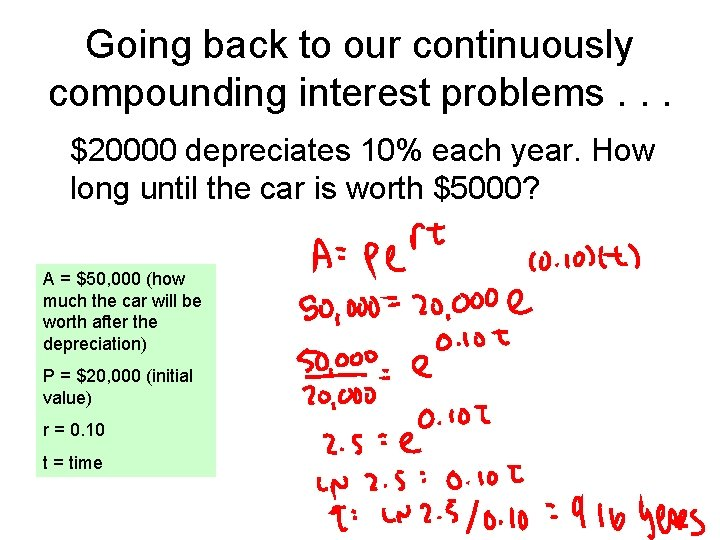Going back to our continuously compounding interest problems. . . \$20000 depreciates 10% each year. How long until the car is worth \$5000? A = \$50, 000 (how much the car will be worth after the depreciation) P = \$20, 000 (initial value) r = 0. 10 t = time